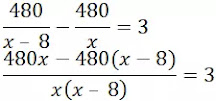# Class10 NCRT Quadratic Equations Exercise – 4.1 pdf || UP Board

1. Check whether the following are quadratic equations:

(i) (x + 1)2 = 2(x – 3)

on simplifying the equation,

(x + 1)2 = 2(x – 3)

x2 + 2x + 1 = 2x – 6

x2 + 7 = 0

Or x2 + 0x + 7 = 0

This equation is of the type ax2 + bx + c = 0.

Hence, the given equation is a quadratic equation.

(ii) x2 – 2x = (-2)(3 – x)

on simplifying the equation,

x2 – 2x = (-2)(3 - x)

x2 – 2x = -6 + 2x

x2 – 4x + 6 = 0

Or x2 – 4x + 6 = 0

This equation is of the type ax2 + bx + c = 0.

Hence, the given equation is a quadratic equation.

(iii) (x – 2)(x + 1) = (x – 1)(x + 3)

on simplifying the equation,

(x – 2)(x + 1) = (x – 1)(x + 3)

x2 – 2x + x – 2 = x2 – x + 3x – 3

-3x + 1 = 0

Or 3x – 1 = 0

This equation is not of the type ax2 + bx + c = 0.

Hence, the given equation is not a quadratic equation.

(iv) (x – 3)(2x + 1) = x(x + 5)

on simplifying the equation,

2x2 – 6x + x – 3 = x2 + 5x

x2 – 10x – 3 = 0

Or x2 +(-10)x + (-3) = 0

This equation is of the type ax2 + bx + c = 0.

Hence, the given equation is a quadratic equation.

(v) (2x – 1)(x – 3) = (x + 5)(x – 1)

on simplifying the equation,

2x2 – x – 6x + 3 = x2 + 5x – x – 5

x2 – 11x + 8 = 0

This equation is of the type ax2 + bx + c = 0.

Hence, the given equation is a quadratic equation.

(vi) x2 + 3x + 1 = (x – 2)2

on simplifying the equation,

x2 + 3x + 1 = x2 – 4x + 4

7x – 3 =0

This equation is not of the type ax2 + bx + c = 0.

Hence, the given equation is not a quadratic equation.

(vii) (x + 2)3 = 2x(x2 – 1)

on simplifying the equation,

x3 + 6x2 + 12x + 8 = 2x3 – 2x

-x3 + 6x2 + 14x + 8 = 0

अर्थात x3 – 6x2 – 14x – 8 = 0

This equation is not of the type ax2 + bx + c = 0.

Hence, the given equation is not a quadratic equation.

(viii) x3 – 4x2 – x + 1 = (x – 2)3

on simplifying the equation,

x3 – 4x2 – x + 1 = x3 – 6x2 + 12x – 8

2x2 – 13x + 9 = 0

2x2 + (– 13)x + 9 = 0

This equation is of the type ax2 + bx + c = 0.

Hence, the given equation is a quadratic equation.

2. Represent the following situations in the form of quadratic equations:

(i) The area of a rectangular plot is 528 m2. The length of the plot (in metres) is one more than twice its breadth. We need to find the length and breadth of the plot.

Solution:

Let the breadth of the plot = x m

Hence, length of plot = 2x + 1 m

Hence, area = x(2x + 1) m2

According to Question,

x(2x + 1) = 528

2x2 + x = 528

2x2 + x – 528 = 0

Hence, the quadratic equation 2x2 + x – 528 = 0 represents the length and breadth of the plot.

(ii) The product of two consecutive positive integers is 306. we need to find the integers.

Let first integer = x

Therefore, second integer = x + 1

So, product = x(x + 1)

According to Question,

x(x + 1) = 306

x2 + x = 306

x2 + x – 306 = 0

Hence, the quadratic equation x2 + x – 306 = 0 represents the product of two consecutive positive integers.

(iii) Rohan’s mother is 26 years older than him. The product of their ages (in years) 3 years from now will be 360. We would like to find Rohon’s present age.

Let Rohan's age = x years

Therefore, age of Rohan's mother = x + 26 years

after three years,

Let Rohan's age = x + 3 years

Therefore, age of Rohan's mother = x + 29 years

Therefore, product of ages = (x + 3)(x + 29) years

According to Question,

(x + 3)(x + 29) = 360

x2 + 3x + 29x + 87 = 360

x2 + 32x – 273 = 0

Hence, the quadratic equation x2 + 32x – 273 = 0 represents the Rohon’s present age.

(iv) A train travels a distance of 480 km at a uniform speed. If the speed had been 8 km/h less, then it would have taken 3 hours more to cover the same distance. We need to find the speed of the train.

Let speed of train = x km/h

Total distance = 480 km

Hence, time taken by the train = 480/x hours

If its speed had been 8 km/m less, then time taken = 480/(x – 8) hours

According to Question,480x – 480x + 3640 = 3(x – 8)x

3640 = 3x2 – 24x

3x2 – 24x – 3640 = 0

Hence, the quadratic equation 3x2 – 24x – 3640 = 0 represents the speed of the train.